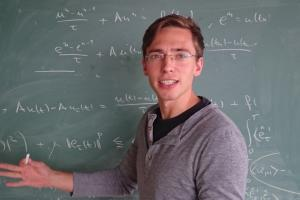Existence Results to the Nonlinear Peridynamic Model in Nonlocal Elastodynamics
Speaker
Mr. Dimitri Puhst
Technical University of Berlin, Germany
Email: puhst@math.tu-berlin.de
Abstract

Peridynamics is a nonlocal elasticity theory based on differences in the deformation instead of the deformation gradient. It is therefore believed to be suitable to describe long range forces as well as material failure. In this talk, we will give an introduction into the theory of peridynamics and consider its equation of motion as a nonlinear second order evolution equation. We present results on existence of weak and measure-valued solutions in the absence of any monotonicity assumption on the peridynamic operator. The method of proof also applies to other nonlocal partial differential equations. This is joint work with Etienne Emmrich.